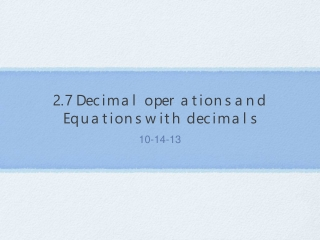DownloadDownload Presentation2.7 Decimal operations and Equations with decimals

# 2.7 Decimal operations and Equations with decimals

Télécharger la présentation## 2.7 Decimal operations and Equations with decimals

- - - - - - - - - - - - - - - - - - - - - - - - - - - E N D - - - - - - - - - - - - - - - - - - - - - - - - - - -
##### Presentation Transcript

1. Review • The rules for performing operations with decimals are the same as those you learned for integers in Ch.1 • For more help with decimal operations (+, - , ÷, x) go to P. 774-776 in the back of your book

2. Adding and subtracting Decimals • -25.38 - (-42.734) = • First rewrite the difference as a sum. Then use the rules for adding numbers with different signs. • -25.38 + 42.734 = 17.354 • -1.3 + (-4.2) = • Use the rule for adding numbers with the same sign. • -5.5

3. Multiplying and dividing decimals • -11.41 ÷ (-0.7) = • Same signs: Quotient is positive • 16.3 • 3.1(-6.8) = • Different signs: Product is negative • -21.08

4. Solving addition and subtraction problems • x + 3.8 = 5.2 • x = 1.4 • Check your solution: 1.4 + 3.8 = 5.2 • 5.2 = 5.2 ✓ • y - 7.8 = 22.3 • y = 30.1 • Check your solution: 30.1 - 7.8 = 22.3 • 22.3 = 22.3 ✓

5. Solving multiplication and division equations • y/11.5 = 0.4 • Multiply each side by 11.5 • y = 4.6 • 7x = 40.6 • Divide each side by 7 • x = 5.8 • Remember to check your solutions ✓

6. Writing and solving an equation • You use an ATM machine to deposit a check for \$122.94 into your savings account. Your receipt from the ATM shows a balance of \$286.59 after the deposit. Find the balance of your savings account before the deposit. • Write and solve an equation to find the balance of your savings account before the deposit. • x = your balance before the deposit • x + 122.94 = 286.59 • To solve the equation, subtract 122.94 from both sides. • x = \$163.59